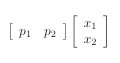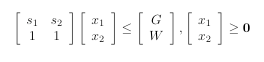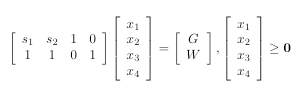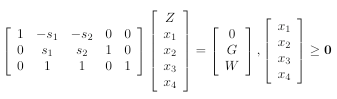# Linear Programming

In my last post on game theory, I said that you could find an optimal
probabilistic grand strategy for any two-player, simultaneous move, zero-sum game.
It’s done through something called linear programming. But linear programming
is useful for a whole lot more than just game theory.

Linear programming is a general technique for solving a huge family of
optimization problems. It’s incredibly useful for scheduling, resource
allocation, economic planning, financial portfolio management,
and a ton of of other, similar things.

The basic idea of it is that you have a linear function,
called an objective function, which describes what you’d like
to maximize. Then you have a collection of inequalities, describing
the constraints of the values in the objective function. The solution to
the problem is a set of assignments to the variables in the objective function
that provide a maximum.

For example, imagine that you run a widget factory, which can
produce a maximum of W widgets per day. You can produce two
different kinds of widgets – either widget 1, or widget 2. Widget one
takes s1 grams of iron to produce; widget 2 needs s2
grams of iron. You can sell a widget 1 for a profit p1 dollars,
and a widget 2 for a profit of p2 dollars. You’ve got G grams of iron
available for producing widgets. In order to maximize your profit, how
many widgets of each type should you produce with the iron you have
available to you?

You can reduce this to the following:

1. You want to maximize the objective function
p1x1 + p2x2, where x1 is the number of type 1 widgets you’ll produce, and x2 is the number
of type 2 widgets.
2. x1 + x2 ≤ W. (You can’t produce more than W widgets.)
3. s1x1 + s2x2 ≤ G. (This is
the constraint imposed by the amount of iron you have available to produce
widgets.)
4. x1 ≥ 0, x2 ≥ 0. (You can’t produce a
negative number of either type of widgets.)

The fourth one is easy to overlook, but it’s really important. One of the tricky things about linear programming is that you need to be sure that you
really include all of the constraints. You can very easily get non-sensical
results if you miss a constraint. For example, if we left that constraint out
of this problem, and the profit on a type 2 widget was significantly higher
than the profit on a type 1 widget, then you might wind up producing a negative number of type 1 widgets, in order to allow you to produce
more than W widgets per day.

Once you’ve got all of the constraints laid out, you can convert the
problem to a matrix form, which is what we’ll use for the
solution. Basically, for each constraint equation where you’ve got
both x1 and x2, you add a row to a coefficient
matrix containing the coefficients, and a row to the result matrix containing the right-hand side of the inequality. So, for example, you’d convert
the inequality “s1x1 + s2x2 ≤ G” to a row [s1,s2] in the coefficient matrix,
and “G” as a row in the result matrix. This rendering of the inequalities
is show below.

Maximize:whereOnce you’ve got the constraints worked out, you need to do something
called adding slack. The idea is that you want to convert the
inequalities to equalities. The way to do that is by adding variables. For
example, given the inequality x1 + x2≤W, you
can convert that to an equality by adding a variable representing
W-(x1+x2): x1+x2+xslack=W, where xslack≥0.

So we take the constraint equations, and add slack variables to all of them,
which gives us the following:

1. Maximize: p1x1 + p2x2
2. x1 + x2 + x3 = W.
3. s1x1 + s2x2 + x4 = G.
4. x1 ≥ 0, x2 ≥ 0.

We can re-render this into matrix form – but the next matrix needs
to includes rows and columns for the slack variables.Now, we need to work the real solution into the matrix. The way that we
do that is by taking the solution – the maximum of the objective function – and naming it “Z”, and adding the new objective function equation into the
matrix. In our example, since we’re trying to maximize the
objective “p1x1 + p2x2“,
we represent that with an equation “Z-p1x1-p2x2=0″. So the
final matrix, called the augmented form matrix of our
linear programming problem is:Once you’ve got the augmented form, there are a variety of techniques that
you can use to get a solution. The intuition behind it is fairly simple: the
set of inequalities, interpreted geometrically, form a convex polyhedron. The
maximum will be at one of the vertices of the polyhedron.

The simplest solution strategy is called the simplex algorithm. In the
simplex algorithm, you basically start by finding an arbitrary vertex
of the polyhedron. You then look to see if either of the edges incident to that point slope upwards. If they do, you trace the edge upward to the next
vertex. And then you look to see if the other edge from that vertex slopes
upwards – and so on, until you reach a vertex where you can’t follow an edge to a higher point.

In general, solving a linear programming problem via simplex is pretty
fast – but it’s not necessarily so. The worst case time of it is
exponential. But in real linear programming problems, the
exponential case basically doesn’t come up.

(It’s like a wide range of problems. There are a lot of problems that are,
in theory, incredibly difficult – but because the difficult cases are
very rare and rather contrived, they’re actually very easy to solve. Two
examples of this that I find particularly interesting are both NP complete.
Type checking in Haskell is one of them: in fact, the general type
inference in Haskell is worse that NP complete: the type validation is
NP-complete; type inference is NP-hard. But on real code, it’s effectively
approximately linear. The other one is a logic problem called 3-SAT. I
once attended a great talk by a guy named Daniel Jackson, talking about
a formal specification language he’d designed called Alloy.
Alloy reduces
its specification checking to 3-SAT. Dan explained this saying: “The bad news
is, analyzing Alloy specifications is 3-SAT, so it’s exponential and NP-complete. But the good news is that analyzing Alloy specifications is 3-SAT,
so we can solve it really quickly.”)

This is getting long, so I think I’ll stop here for today. Next post, I’ll
show an implementation of the simplex algorithm, and maybe talk a little bit
about the basic idea behind the non-exponential algorithms. After that, we’ll
get back to game theory, and I’ll show how you can construct a linear
programming problem out of a game.

## 0 thoughts on “Linear Programming”

1.Jon Raphaelson

You know, I think that in just 5 or so paragraphs you elucidated that more than the week and a half we spent during my undergrad algorithms class.
My hit tips to you, sir.

2.Canuckistani

Indeed, this post is a gem of clarity.

3.Kalle Svensson

Nice introduction! A small typo: on the first row of the augmented matrix, you have -s_1 & -s_2 instead of -p_1 & -p_2.

4.Joao Natali

Nice post!
May I suggest in your next post the inclusion of a paragraph about Mixed Integer Linear Programming? The concept follows easily from LP, and MILP problems are, in my opinion, even more interesting.

5.Bluefer

I believe your link to Alloy wasn’t intended to be recursive, and this http://alloy.mit.edu/ is the correct link.

6.Jarmo Jomppanen

Daniel Jackson the archaeologist?
Nicely done. There is something in your way of writing that makes things immediately come through.

7.Jim Battle

You say, in support of constraint 4, that producing negative type 1 widgets is nonsensical. Actually, it might make sense. If you have an inventory of type 1 widgets, it may be worth it to melt them down so you can produce more of type 2 widgets.

8.Tony

Rocking post!

9.Steve P.

Great post. Duality might be a good “next topic” that I’d really be interested in reading.

10.Alok Bakshi

Hi Mark,
Thanks for the nice introduction of Linear programming.
May I add one more subtle point here. Regarding your example of widget factory, I think x1 and x2 (number of type1/type2 widgets) should be defined as integer variables. Truncation of integer variables in ‘optimal solution’ is not a good idea either since it might result in an infeasible or sub-optimal solutions.

11.Philip Løventoft

Hi Mark
I’ve been thinking for a while about how your posts are usually much clearer than most textbooks. Have you considered writing a book, for example about Haskell? I think a lot of people would buy it.
Anyway I read your blog with much pleasure, including this post, keep up the good work 🙂

12.timg

why is it always widgets?????

13.Doug

Hi Mark,
Good post on linear programming.
Doesn’t there exist a condition such that the Dynamic Programming of Richard Bellman becomes more efficient?

14.Ian

“why is it always widgets?”
Especially the iron ones! They’re like, so five minutes ago.
One word: Plastics!

15.nick

Nice article. I was lightly exposed to the the simplex method when I was looking into the downhill simplex method or Nelder-Mead method. It was easy to spot the difference, but it did make finding what I needed a bit harder.
I can’t wait to see the rest. keep it up

16.wk

I second Alok Bakshi’s request (@ #10) for more info on how integer-valued data affect this.

17.Sili

I think I understood this better than in my linear algebra class … eight years ago, I think.
I probably came up in the numerical analysis course too, but I was singularly unmotivated for learning anything to do with programming back then. I put off taking those two mandatory computerscience classes for as long as possible.
Obviously I feel quite stupid now. I’ve even had a few problems that might have been easily solvable with a litte bit of programming knowledge.
I’ve lost my small programmes from back then due to a harddisk failure and a theft, so I couldn’t just copy the bits I’d written (/copied from the blackboard) to handle exceptions when reading in a file – which was what I needed. And somehow I’ve never found the impetus to try to relearn anything.
Can anyone recommend some good ressources à la The Utter Idiot’s Guide to Java and Python? Something online with interactive tutorials that does *not* assume that the reader/learner knows *anything*.

18.Kalle Svensson

wk: Well, integer valued variables wreak havoc on the structure of the feasible region. With integrality constraints, it is no longer a convex polyhedron but rather a disjunct gathering of points. This makes it impossible to use the simplex method and other linear programming methods as designed. Generally, a linear problem becomes *much* harder when integrality constraints are added.
Integer linear programs can be solved for example by a method of tree searching using LP relaxations (that is the same problem with removed integrality constraints) to establish bounds on the optimal solution. This is called Branch and bound. There are other methods for solving general ILPs and MILPs, but many successful methods are also problem dependent.

19.Hephaestus

Mark –
I knew that if I hung on through your set theory postings, you’d finally get around to topics that I know something about! Thanks for taking the time to write about these subjects. As a physics major, I had way too much college and graduate math, most of which didn’t stick very well, but reading your posts helps to shake some of the dust off.
One consideration of linear programming that gets shorted in most treatments is that real world problems are often over-determined. As an example, in the distant past I wrote a program that determined the composition of piles of scrap metal based upon the analysis of the metal that resulted when various quantities of each were mixed together and melted. Since good scrap costs more than cheap scrap, the idea was to produce the least cost load that would produce the designed final chemistry. The problem is that the chemistry of the scrap piles themselves are unknown.
Many of the standard LP algorithms fall down badly in these conditions.

20.Mark C. Chu-Carroll

Alok:
You’re jumping ahead of me 🙂
The problem, as formulated in this post, is actually incorrect, as you’ve pointed out. But I wanted to work up to that, by first showing simplex, and then pointing out on how when you instantiated the problem, you’d get answers telling you to make 3.5 of widget 1, and 2.7 of widget 2.
If you constrain the solution to integers, then you get a slightly different problem called integer linear programming. That little change has a huge impact. Integer linear programming is hard. Really hard. The decision-function formulation of it is NP-complete; computing the actual solution is NP-hard. In general, we either find cases of it where there’s a relatively small number of variables (in which case the exponential factor doesn’t make it non-computable), or we use heuristics to find nearly optimal solutions. As far as anyone knows, there’s no fast way to compute a true optimal solution to an integer linear programming in polynomial time.

21.wk

Great — looking forward to it.

22.Al

Don’t forget to finish this…

23.GB

The first matrix with slack variables should be s1, s2, 0 , 1 & 1, 1, 1, 0

24.Philippe

Just a small comment about NP-completeness: the fact that a problem reduces to 3SAT doesn’t prove that it is NP-complete (2SAT for instance :p), the reduction is the other way round (see also your post of July 15, 2007).
And I don’t know if you’re familiar with FPT (fixed-parameter tractable) algorithms, but it’s a pretty nice example on how to deal with some NP-complete problems: find an algorithm whose complexity is a polynomial in n times some function exponential in k, where k is a small parameter in practice.

25.ping

can you include about duality next time?